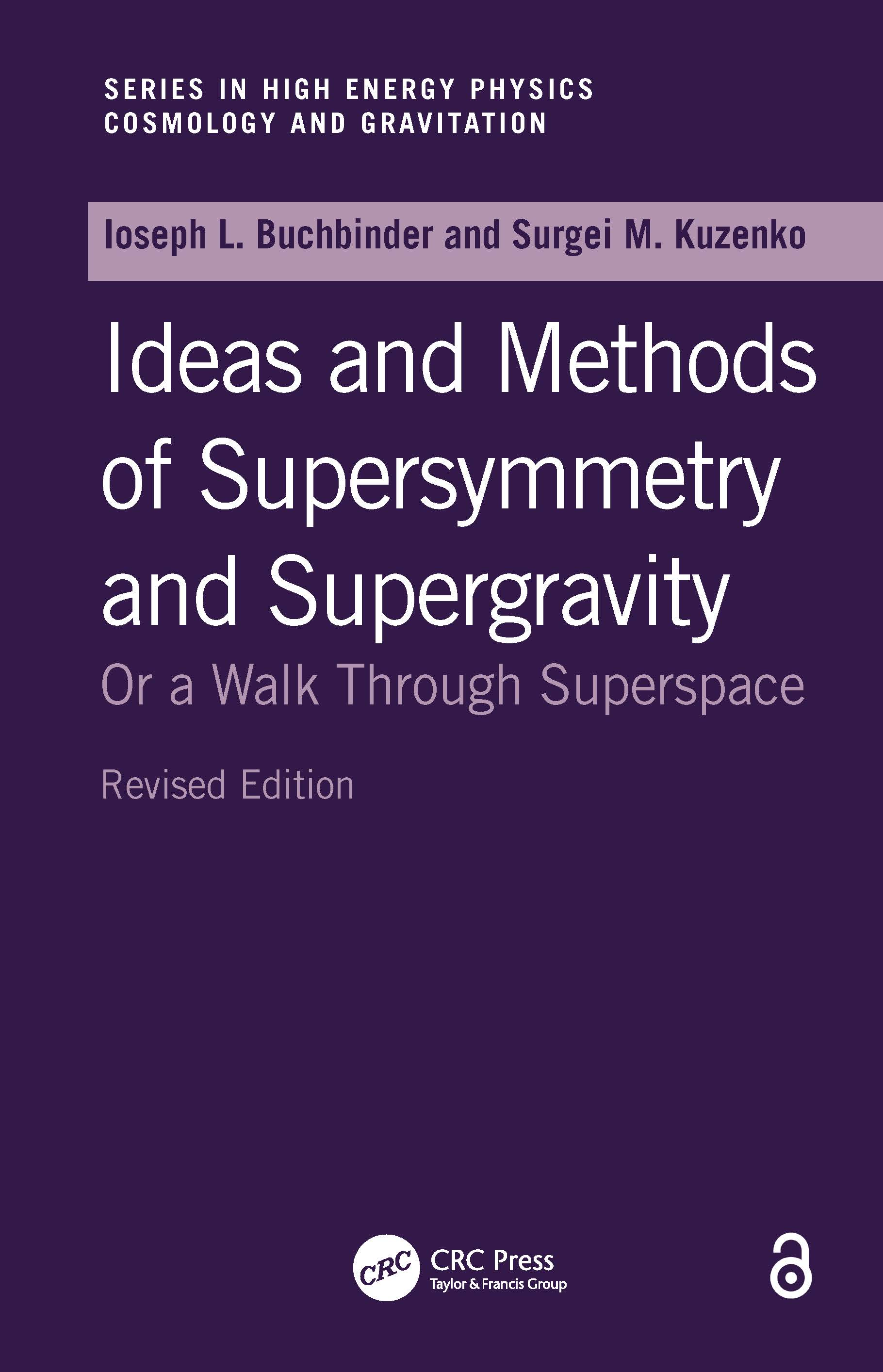# Introduction to Supersymmetric Field Theory

## 1st Edition

CRC Press

656 pages

##### Purchasing Options:\$ = USD
Hardback: 9780750305068
pub: 1998-01-01
\$120.00
x

FREE Standard Shipping!

### Description

Ideas and Methods of Supersymmetry and Supergravity: Or a Walk Through Superspace provides a comprehensive, detailed, and self-contained account of four dimensional simple supersymmetry and supergravity. Throughout the book, the authors cultivate their material in detail with calculations and full discussions of the fundamental ideas and motivations. They develop the subject in its superfield formulations but where appropriate for illustration, analogy, and comparison with conventional field theory, they use the component formulation. The book discusses many subjects that, until now, can only be found in the research literature. In addition, it presents a plethora of new results.

Combining classical and quantum field theory with group theory, differential geometry, and algebra, the book begins with a solid mathematical background that is used in the rest of the book. The next chapter covers algebraic aspects of supersymmetry and the concepts of superspace and superfield. In the following chapters, the book presents classical and quantum superfield theory and the superfield formulation of supergravity. A synthesis of results and methods developed in the book, the final chapter concludes with the theory of effective action in curved superspaces.

After studying this book, readers should be well prepared to pursue independent research in any area of supersymmetry and supergravity. It will be an indispensable source of reference for advanced graduate students, postdoctoral faculty, and researchers involved in quantum field theory, high energy physics, gravity theory, mathematical physics, and applied mathematics.

### Reviews

"The authors are among the most knowledgeable in this field…"

-Physics Today

"The student who wants to learn the subject as it is practiced by superspace afficionados will find here just about everything he/she needs … The experts will find here much useful reference material that is only accessible by hunting through journals … The book is highly recommended."

-General Relativity and Gravitation

Preface. Mathematical background: The Poincar^D'e group, the Lorentz group; Finite-dimensional representations of ^ISpin(3,1); The Lorentz algebra; Two-component and four-component spinors; Representations of the Poincar^D'e group; Elements of differential geometry and gravity; The conformal group; The mass-shell field representation; Elements of algebra with supernumbers; Elements of analysis with supernumbers; The supergroup of general coordinate transformations on R^Tp/q. Supersymmetry and superspace: Introduction: from R^Tp/q to supersymmetry; Superalgebras, Grassmann-shells and super Lie groups; The Poincar^D'e superalgebra; Unitary representation of the Poincar^D'e superalgebra; Real superspace R^T4/4 and superfields; Complex superspace C^T4/2, chiral superfields and covariant derivatives; The on-shell massive superfield representations; The on-shell massless superfield representations; From superfields to component fields; The superconformal group. Field theory in superspace: Supersymmetric field theory; Wess-Zumino model; Supersymmetric nonlinear sigma-models; Vector multiplet models; Supersymmetric Yang-Mills theories; Geometric approach to super Yang-Mills theories; Classically equivalent theories. Quantized superfields: Picture-change operators; Equivalence of component field and superfield perturbation theories; Effective action (super) funtional; The Wess-Zumino model: perturbative analysis; Note about gauge theories; Feynman rules for super Yang-Mills theories; Renormalization; Examples of counterterm calculations: an alternative technique; Superfield effective potential. Superspace geometry of supergravity: Gauge group of supergravity and supergravity fields; Superspace differential geometry; Supergeometry with conformal supergravity constraints; Prepotentials; Einstein supergravity; Prepotential de formations; Supercurrent and supertrace; Supergravity in components. Dynamics in supergravity: Pure supergravity dynamics; Linearized supergravity; Superg

### Subject Categories

##### BISAC Subject Codes/Headings:
SCI005000
SCIENCE / Astrophysics & Space Science
SCI051000
SCIENCE / Nuclear Physics
SCI055000
SCIENCE / Physics